# Numerical Methods: Runge–Kutta Algorithms

September 10, 2013 —Comments Off

As part of our ongoing plan to expand Wolfram|Alpha’s numerical method functionality to more kinds of algorithms, we recently addressed solving differential equations. There are a number of different numerical methods available for calculating solutions, the most common of which are the Runge–Kutta methods. This family of algorithms can be used to approximate the solutions of ordinary differential equations.

The Runge–Kutta methods are iterative ways to calculate the solution of a differential equation. Starting from an initial condition, they calculate the solution forward step by step. The most common method is the fourth-order Runge–Kutta method, often simply referred to as the Runge–Kutta method. With this algorithm we can solve differential equations such as y‘ = -2xy, y(0) = 2: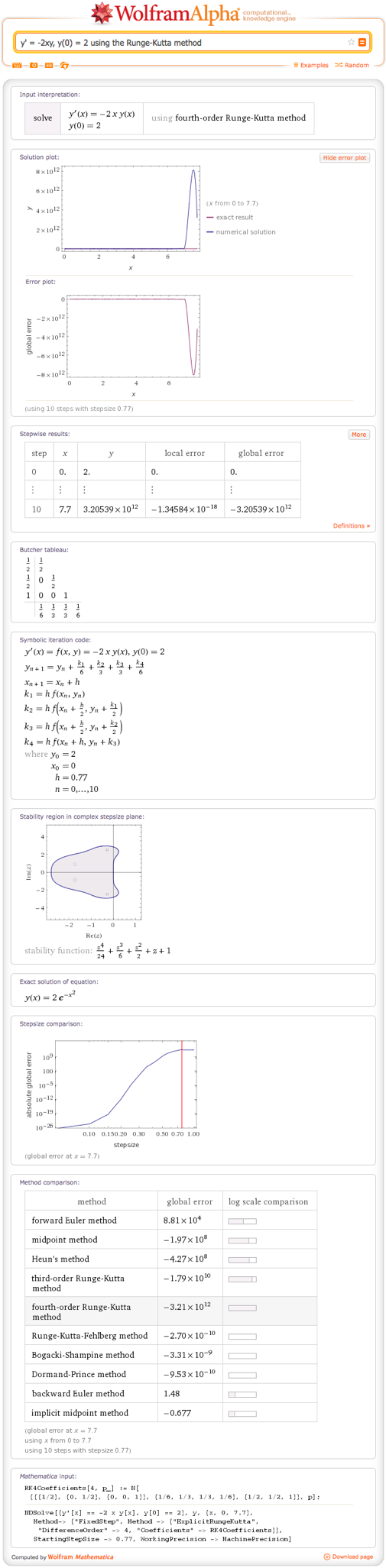You can specify the range (Runge–Kutta method y‘ = – y, y(1) = 3, from = 1, 5) and the step size (use the rk4 method to solve y‘ = – x with step size 0.25) and solve higher-order differential equations (solve y” = -2+ 4x^2y‘ with the fourth-order Runge–Kutta algorithm).

If we look at the symbolic form for the Runge–Kutta method (symbolic form for the Runge–Kutta method, y’ = -2xy, y(0) = 2, from 1 to 3, h = .25), we can see that each step of the solution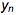is determined by feeding results of the prior step into the differential equation.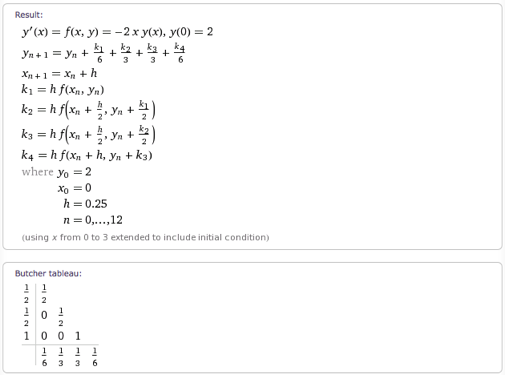The major difference between the various Runge–Kutta methods can be found in the Butcher tableau. This is a shorthand way of writing out the coefficients that go into the process above. The values in the left-hand column are the coefficients for the step size h added to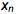. Those in the bottom row are the coefficients for the terms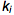. The matrix of values is used to determine the coefficients for the termsadded to the second argument of f.

One of the major divisions among the Runge–Kutta methods is between the explicit and implicit methods. The fourth-order Runge–Kutta method shown above is an example of an explicit method. One problem with explicit methods is their limited stability, which can be an issue with stiff calculations such as partial differential equations. In such cases, an implicit method such as the implicit midpoint method ({y'(x) = -2 y, y(0)=1} from 0 to 2 by implicit midpoint) may be preferable: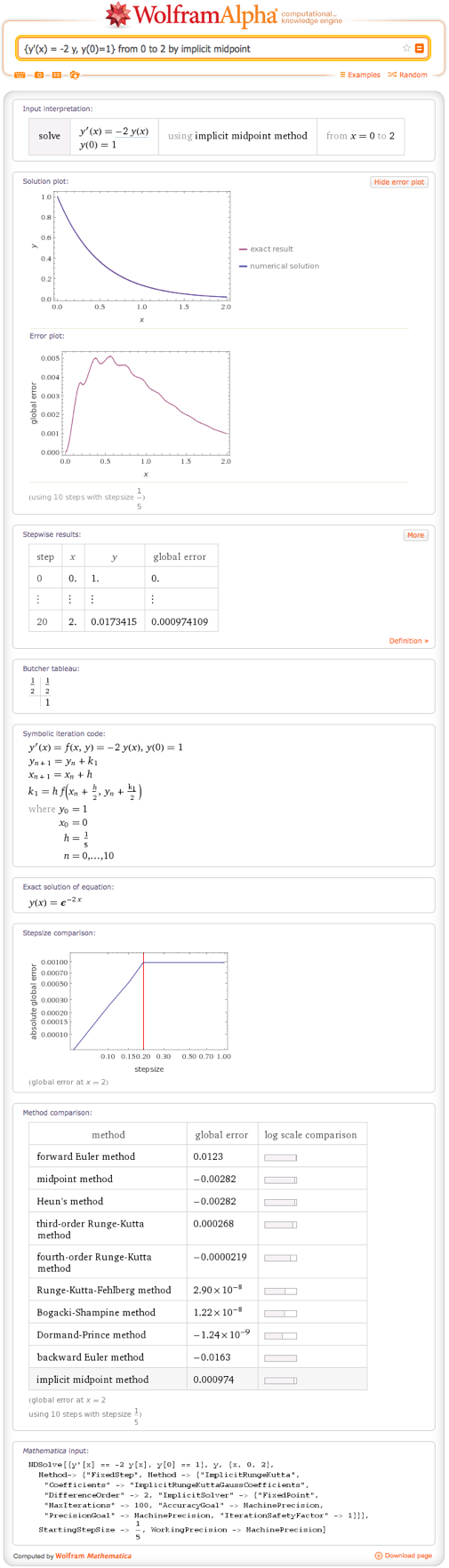We can see that the implicit midpoint method, a second-order method, gives a global error much smaller than either Heun’s method or the explicit midpoint method, which are both explicit second-order methods.

As we can see from the Butcher tableau, implicit methods use all matrix values, not just the lower-left triangle. This makes them more complicated to calculate, since a series of algebraic equations must be solved at each step. Another option for improvement on explicit methods is to adjust the step size at each iteration. This is supported by adaptive methods such as the Bogacki–Shampine algorithm ({y'(x) = -2 y, y(0)=1} from 0 to 10 by Bogacki–Shampine method). Looking at the Butcher tableau for this method, we can see that a second row has been added to the bottom. This set of coefficients is used at each step to evaluate the accuracy of the calculation and adjust the step size accordingly: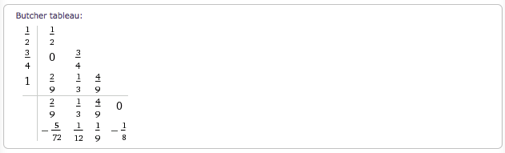We plan to continue to expand Wolfram|Alpha’s numerical method functionality to more kinds of algorithms. We hope you enjoy exploring the numerical solutions to differential equations, and we look forward to bringing more such functionality to you in the future.

1 Comment

Dear sir

I have found your code better. I need a code for system of fractional differential equation initial value problem by Runge-Kutta method for biological SIR model.

Posted by Muhammad Altaf Khan September 16, 2014 at 6:35 am